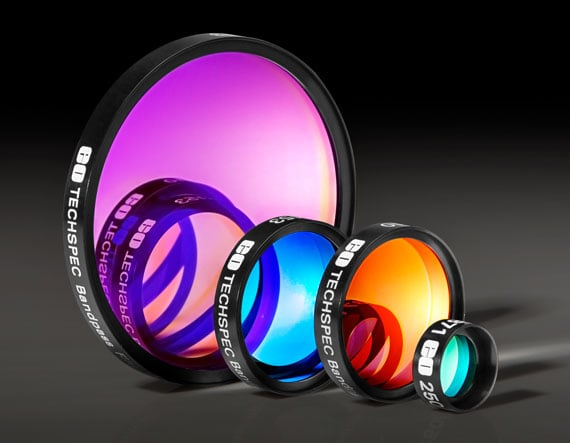Edmund Optics uses cookies to optimise and enhance the features and content on our website. By continuing to use this website, you consent to our use of cookies.

# Laser Induced Damage Threshold Scaling Calculator

The laser induced damage threshold (LIDT) of an optical component is dependent on wavelength, pulse duration, and beam diameter. If an optic’s specified LIDT is at a different wavelength, pulse duration, or diameter than that of the application, the LIDT must be re-evaluated at the application specifications. For small changes in these specifications, the resulting scaling of LIDT can be approximated. The larger the difference between the test conditions and the use conditions, the less accurate the scaling. This calculator takes a given LIDT value for a pulsed laser at a known wavelength (λ1), pulse duration (τ1), and beam diameter (∅1), and scales it to a new LIDT at a different wavelength (λ2), pulse duration (τ2), and beam diameter (∅2).

### Beam Diameter 2, ∅2(mm):

Note: This calculator is valid for wavelengths between 248-1100nm, pulse durations between 1-100ns, and beam diameters between 1-25mm. Since the equation used is only valid for small shifts in use parameters, this calculator is only intended to be used for shifts in use conditions within these limits:
• ± 5% of input wavelength
• ± factor of 3 of pulse duration
• ± factor of 2 of beam diameter## Equations and Corresponding Legend:

$$\text{LIDT} \! \left( \lambda_2, \tau_2, ∅_2 \right) \approx \text{LIDT} \! \left( \lambda_1, \tau_1, ∅_1 \right) \times \left( \frac{\lambda_2}{\lambda_1} \right) \times \sqrt{\frac{\tau_2}{\tau_1}} \times \left( \frac{∅_1}{∅_2} \right)^2$$
 λ1 Wavelength of known LIDT λ2 Wavelength of new LIDT τ1 Pulse duration of known LIDT τ2 Pulse duration of new LIDT ∅1 Beam diameter of known LIDT ∅2 Beam diameter of new LIDT

## Example

Question: What is the approximate LIDT of an optic at a wavelength of 515nm, pulse duration of 30ns, and beam diameter of 2mm if the optic has a specified LIDT of 10 J/cm2 at a wavelength of 532nm, pulse duration of 20ns, and beam diameter of 3mm?

Answer: The approximate LIDT at the new use conditions can be determined by:

\begin{align}
\text{LIDT} \! \left( 515 \text{nm}, 30 \text{ns}, 2 \text{mm} \right) &  \approx 10 \tfrac{\text{J}}{\text{cm}^2} \times \left( \frac{515 \text{nm}}{532 \text{nm}} \right) \times \sqrt{\frac{30 \text{ns}}{20 \text{ns}}} \times \left( \frac{3 \text{mm}}{2 \text{mm}} \right)^2  \\
& = 26.7 \tfrac{\text{J}}{\text{cm}^2}
\end{align}

The approximate LIDT at the new use conditions will be more than 2X higher than the LIDT at the test conditions.

## Related Resources and Products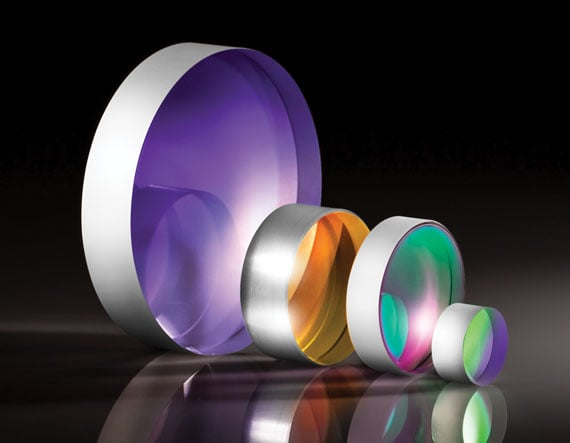### Laser LineMirrors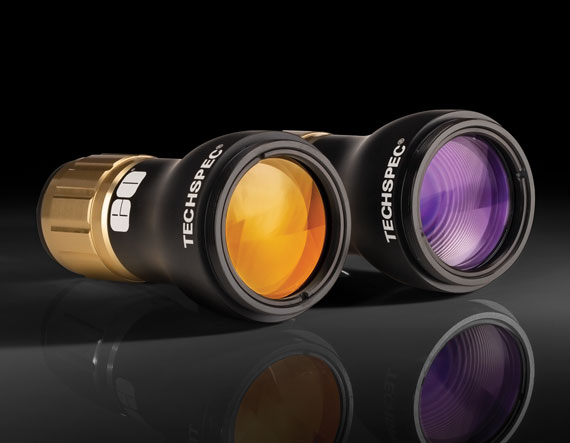### Laser BeamExpanders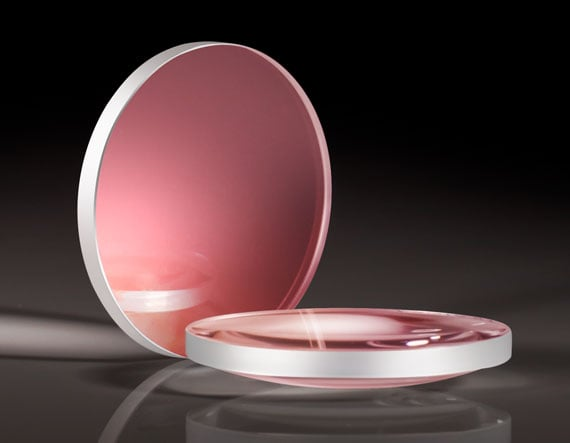### LaserLenses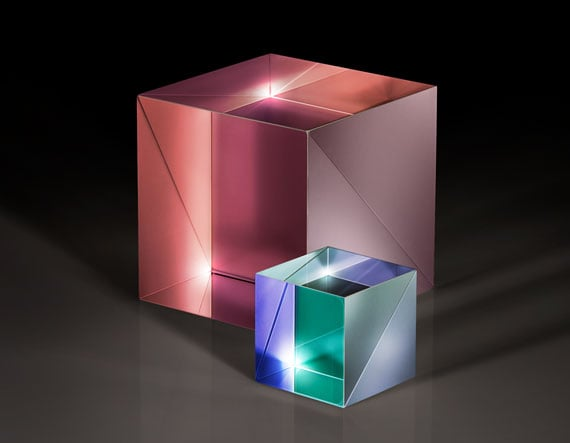### LaserBeamsplitters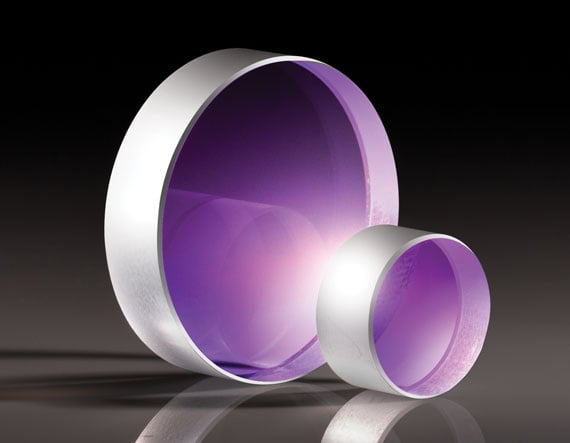### LaserWindows### LaserPolarization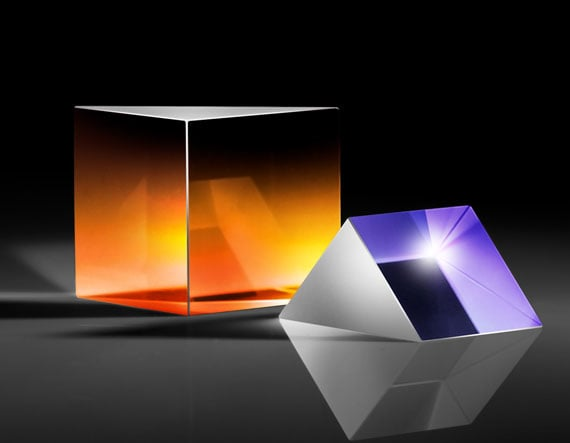### LaserPrisms